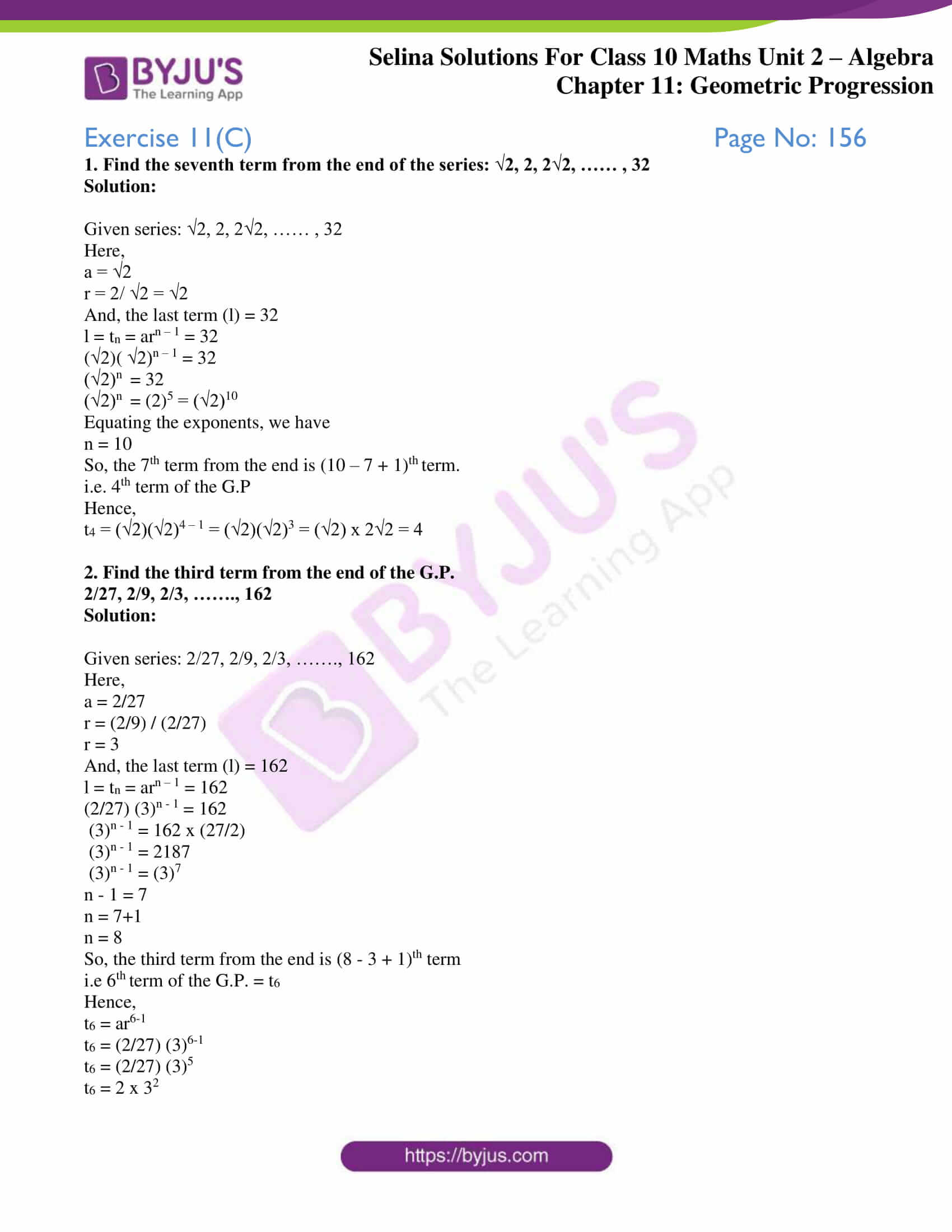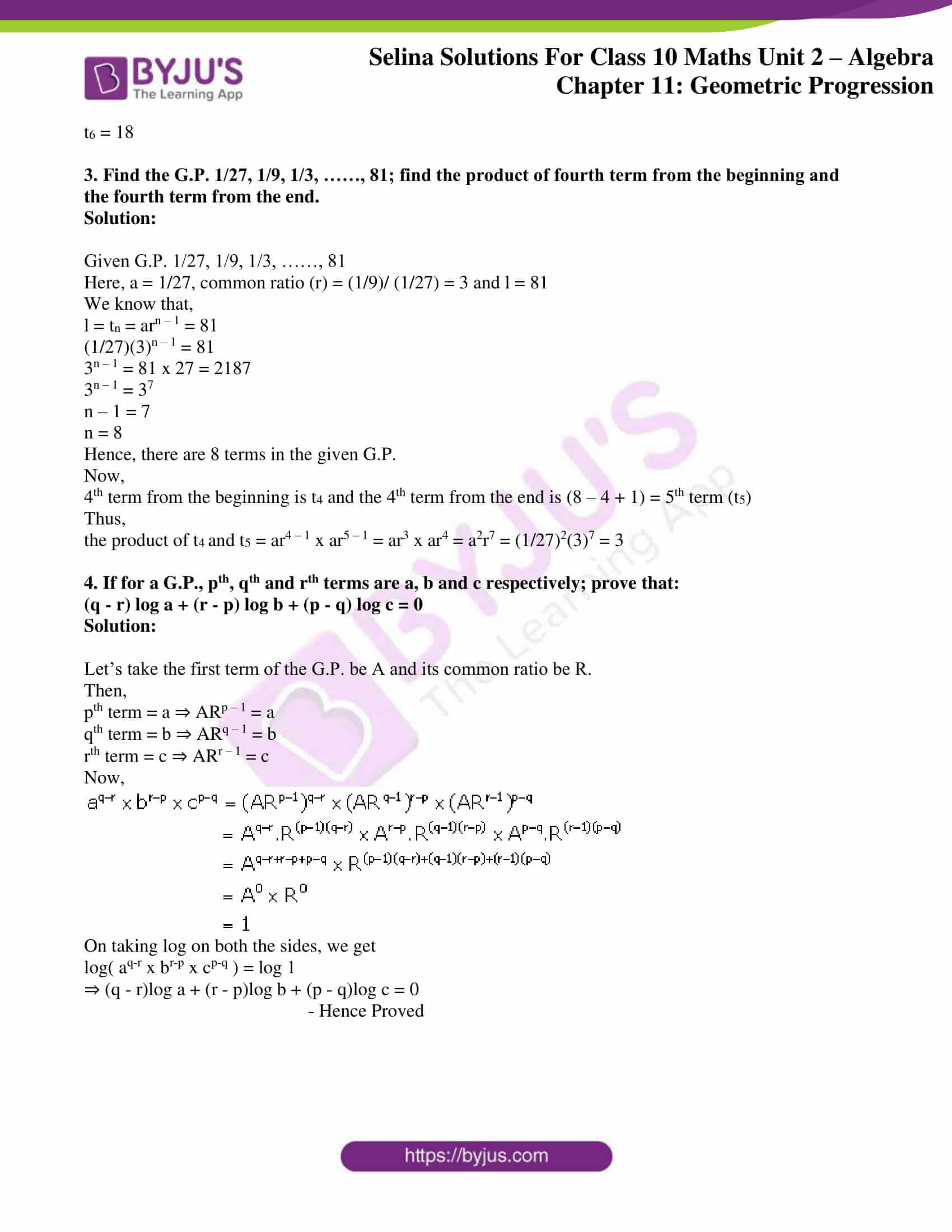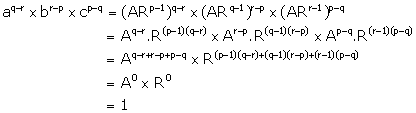# Selina Solutions Concise Maths Class 10 Chapter 11 Geometric Progression Exercise 11(C)

Properties of a G.P. are discussed in this exercise. Students having any doubts in understanding any concept of ICSE Class 10 Mathematics can refer to the Selina Solutions for Class 10 Maths. The solutions are created by subject matter experts having wide experience in the academic field. Also, access accurate answers to this exercise in the Selina Solutions Concise Maths Class 10 Chapter 11 Geometric Progression Exercise 11(C) PDF, available in the links provided below.

## Selina Solutions Concise Maths Class 10 Chapter 11 Geometric Progression Exercise 11(C) Download PDF### Access Selina Solutions Concise Maths Class 10 Chapter 11 Geometric Progression Exercise 11(C)

#### Exercise 11(C) Page No: 156

1. Find the seventh term from the end of the series: √2, 2, 2√2, …… , 32

Solution:

Given series: √2, 2, 2√2, …… , 32

Here,

a = √2

r = 2/ √2 = √2

And, the last term (l) = 32

l = tn = arn – 1 = 32

(√2)( √2)n – 1 = 32

(√2)n = 32

(√2)n = (2)5 = (√2)10

Equating the exponents, we have

n = 10

So, the 7th term from the end is (10 – 7 + 1)th term.

i.e. 4th term of the G.P

Hence,

t4 = (√2)(√2)4 – 1 = (√2)(√2)3 = (√2) x 2√2 = 4

2. Find the third term from the end of the G.P.

2/27, 2/9, 2/3, ……., 162

Solution:

Given series: 2/27, 2/9, 2/3, ……., 162

Here,

a = 2/27

r = (2/9) / (2/27)

r = 3

And, the last term (l) = 162

l = tn = arn – 1 = 162

(2/27) (3)n – 1 = 162

(3)n – 1 = 162 x (27/2)

(3)n – 1 = 2187

(3)n – 1 = (3)7

n – 1 = 7

n = 7+1

n = 8

So, the third term from the end is (8 – 3 + 1)th term

i.e 6th term of the G.P. = t6

Hence,

t6 = ar6-1

t6 = (2/27) (3)6-1

t6 = (2/27) (3)5

t6 = 2 x 32

t6 = 18

3. Find the G.P. 1/27, 1/9, 1/3, ……, 81; find the product of fourth term from the beginning and the fourth term from the end.

Solution:

Given G.P. 1/27, 1/9, 1/3, ……, 81

Here, a = 1/27, common ratio (r) = (1/9)/ (1/27) = 3 and l = 81

We know that,

l = tn = arn – 1 = 81

(1/27)(3)n – 1 = 81

3n – 1 = 81 x 27 = 2187

3n – 1 = 37

n – 1 = 7

n = 8

Hence, there are 8 terms in the given G.P.

Now,

4th term from the beginning is t4 and the 4th term from the end is (8 – 4 + 1) = 5th term (t5)

Thus,

the product of t4 and t5 = ar4 – 1 x ar5 – 1 = ar3 x ar4 = a2r7 = (1/27)2(3)7 = 3

4. If for a G.P., pth, qth and rth terms are a, b and c respectively; prove that:

(q – r) log a + (r – p) log b + (p – q) log c = 0

Solution:

Let’s take the first term of the G.P. be A and its common ratio be R.

Then,

pth term = a ⇒ ARp – 1 = a

qth term = b ⇒ ARq – 1 = b

rth term = c ⇒ ARr – 1 = c

Now,On taking log on both the sides, we get

log( aq-r x br-p x cp-q ) = log 1

⇒ (q – r)log a + (r – p)log b + (p – q)log c = 0

– Hence Proved

### Access other exercises of Selina Solutions Concise Maths Class 10 Chapter 11 Geometric Progression

Exercise 11(A) Solutions

Exercise 11(B) Solutions

Exercise 11(D) Solutions